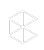Learn from the best and brightest in legal, technology, and beyond! Join us at the 2019 Clio Cloud Conference — San Diego | October 21-22, 2019.

# What is the Difference Between Simple Interest and Compound Interest?

Objective:

• Simple interest and compound interest explained

Environment:

• Clio Web App

Additional Information:

n/a

Answer:

• Simple interest is calculated based on the principal amount (the initial amount charged to the client).
• Simple Interest=(Principle) x (Interest Rates) x (Time)
• Compound interest is calculated on the principle plus any interest accrued on the Bill.
• Compound Interest= Principal x (1+Interest)Number of Periods - Principal
Was this article helpful?
This information is confusing or wrong
This isn't the information that I was looking for
I don't like this functionality